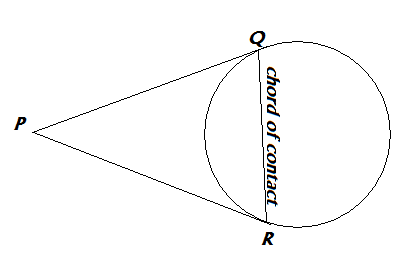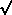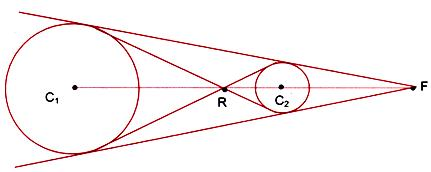### Circles - Tutorial, Solved Problems, MCQ Quiz/Worksheet - The Equation in Regular/Parametric form, Tangents and Normals, Families

Target Audience: High School Students, College Freshmen and Sophomores, Class 11/12 Students in India preparing for ISC/CBSE and Entrance Examinations like the IIT-JEE

, and anyone else who needs this Tutorial as a reference!

After reading this tutorial you might want to check out some of our other Mathematics Quizzes as well.
 Quizzes on ProgressionsMCQ #1: Arithmetic Progression MCQ #2: Geometric ProgressionMCQ #3 : More on Geometric Progressions.MCQ #4 : Harmonic Progressions. MCQ #5: More on Harmonic ProgressionMCQ #6: Mixed ProgressionsComplex NumbersMCQ #1MCQ #2: More on Complex NumbersQuadratic EquationsMCQ Quadratic EquationsQuadratic In-equationsMCQ Quadratic In-equations Coordinate Geometry - Straight LinesMCQ #1: Cartesian Planes, Straight Line BasicsMCQ #2 on Straight LinesMCQ #3 on Straight LinesMCQ #4 on Straight LinesCircles1 MCQ #1 on Circles. 2 MCQ #2 on Circles. 3 MCQ #3 on Circles. Conic Sections- Parabola, Hyperbola, Ellipse1 MCQ- The Basics of Conic Sections2 MCQ on Parabola..3 MCQ on Hyperbola4 MCQ on Ellipses. ProbabilityMCQ #1 on Basic ProbabilityMCQ #2: More Challenging Problems on ProbabilityMCQ #3- Conditional Probability and Bayes Theorem

The Circle
The Equation in Standard and Parametric Forms, Chords, Diameters, Tangents### Tangents to a Circle - Pairs of Tangent from an External Point, Chord of Contact, Normal

• T, S, S1: If x2+y2+2gx+2fy+c=0 is the equation of the circle, then S= x2+y2+2gx+2fy+c, S1= x12+y12+2gx1+2fy1+c (putting some point (x1,y1) in the equation) and T=xx1+yy1+g(x+x1)+f(y+y1).
• A pair of tangents from an external point P(x1,y1) is SS1=T2
• The chord of contact 1,y1) is T=0.• A chord with mid point (x1,y1) is T=S1
• Tangent:
• Equation of tangentT=0 where (x1,y1) is the point of contact.
• Length of Tangent drawn from (x1,y1) is √S1
• For x2+y2=a2
• y = mx ± a[1 +m²]

#### Normal: The normal is a line perpendicular to the tangent at the point of contact. It always passes through the centre of the circle.

• Conditions for Two circles with centres C1(x1, y1) and C2(x2, y2) and radii r1, r2 respectively, touch each other.  (i) Internally: If |C1 C2| = |r2 – r1(ii) Externally: If |C1 C2| = |r2 – r1|

### Common Tangents - Direct and Transverse(i) The direct common tangents to two circles meet on the line of centres and divide it externally in the ratio of the radii.(ii) The transverse common tangents also meet on the line of centres and divide it internally in the ratio of the radii.• When one circle lies completely inside the other without touching, there is no common tangent.
• When two circles touch each other internally 1 common tangent can be drawn to the circles.
• When two circles intersect in two real and distinct points, 2 common tangents can be drawn to the circles.
• When two circles touch each other externally, 3 common tangents can be drawn to the circles.
• When two circles neither touch nor intersect and one lies outside the other, then 4 common tangents can be drawn.

## Family of Circles

(i) If S is x2 + y2 + 2gx + 2fy + c=0 and S’ is x2 + y2 + 2g’x + 2f’y + c=0 are two intersecting circles, then S + λS’ = 0, λ ≠ –1, is the equation of a family of circles passing through the points of intersection of S = 0 and S’ = 0.
2 + y2 + 2gx + 2fy + c = 0 is a circle which is intersected by the straight line L as ax + by + c = 0 in two real and distinct points, then S + λL = 0 is the equation of a family of circles passing through the points of intersection of S = 0 and L = 0.
1 = m (x – x1) at (y1, x1) for any values of m is (x – x1)2 + (y – y1)2 + λ[(y – y1) – m(x – x1)] = 0.
.

### Parametric Equation of a Circle:

x = a + r * cos A
y = b + r * sin A

Where 0 <= A <= (2*PI) radians

#### Here are some of the problems solved in this tutorial :

Q:

Q:Find all the common tangents to the circles x2 + y2 – 2x – 6y + 9 = 0 and x2 + y2 – 6x – 2y + 1 = 0.

Q:Find all the common tangents to the circles x2 + y2 – 2x – 6y + 9 = 0 and x2 + y2 – 6x – 2y + 1 = 0.

Q:Find the equation of the circle which passes through two points of the x axis which are at a distance of 4 from the origin and whose radius is 5.

Q:A circle of radius 5 units touches the coordinate axes in the first quadrant. If the circle makes one complete roll on x axis along the positive direction, find its equation in new position.

Q:Find the equation of circle whose radius is 5 and which touches the circle x2+y2-2x-4y-20=0 externally at the point (5,5).

Q:A circle of radius 2 lies n the first quadrant touching both axes. Find the equation of the circle centred at (6,5) and touching the above circle externally.

Q:Find the equation of the circle which touches the coordinate axes and whose centre lies on the line x-2y=3.

Q:If the point (2,k) lies outside the circles x2+y2+x-2y-14=0 and x2+y2=13, then what are the values that k can take?

Q:Find the equation of the incircle formed by the coordinate axes and the line 4x+3y=6.

Q:Find the equation of circle which touches the lines 4x-3y+10=0 and 4x-3y-30=0 and whose centre lies on the line 2x+y=0.

Q:Let S be any circle with centre (0,√2). Prove that at most 2 rational points can be there on S.

Q:The straight line x/a + y/b =1 cuts the coordinate axes at A and B respectively. Find the equation of the circle passing through A, B, and O(0,0).

Q:Show that the points (9,1), (7,9), (-2,12), (6,10) are concyclic.

Q:On the line joining (1,0) and (3,0) an equilateral triangle is drawn, having its vertex in the first quadrant. Find the equation to the circles described on its sides as diameter.

Q:Find the area of equilateral triangle inscribed in x2+y2+2gx+2fy+c=0.

Q:Write the equation of normal to x2 + y2 = 25 at (3, 4)?

Q:Find a circle passing through the intersection of x+ y2 - 4 = 0 and x2 + y2 - 6x + 5 = 0 which passes through the point (2, 1)?

Q:Find the equation of the circle described on the common chord of the circles x+ y2 – 4x – 5 = 0 and x2 + y2 + 8y+ 7 = 0 as diameter

Q:Find the co-ordinates of the point from which tangents are drawn to the circle x2 + y2– 6x – 4y + 3= 0 such that the mid-point of its chord of contact is (1, 1).

Q:When do two circles intersect orthogonally?

Q:Find all the common tangents to the circles x2 + y2 – 2x – 6y + 9 = 0 and x2 + y2 – 6x – 2y + 1 = 0.

### Equation of a Circle:

The circle is a locus of points, equidistant from a given point.

Where (a,b) is the center of the circle and r is the radius of the circle.

## MCQ Quiz #1 on Circles.

Answers will be e-mailed to the id which you fill up.